September 18, 2018

Workout

Question 1(a)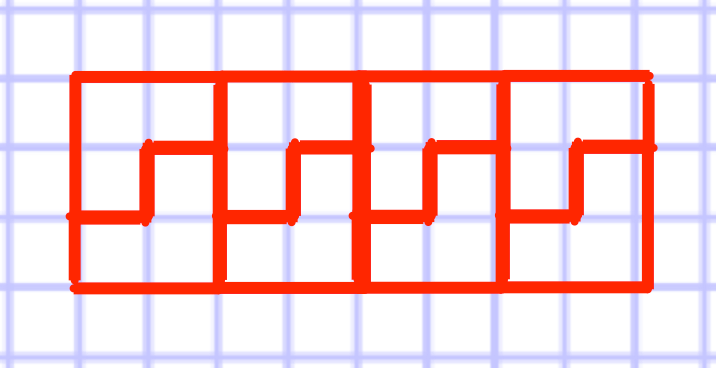Question 1(b)Question 1(c)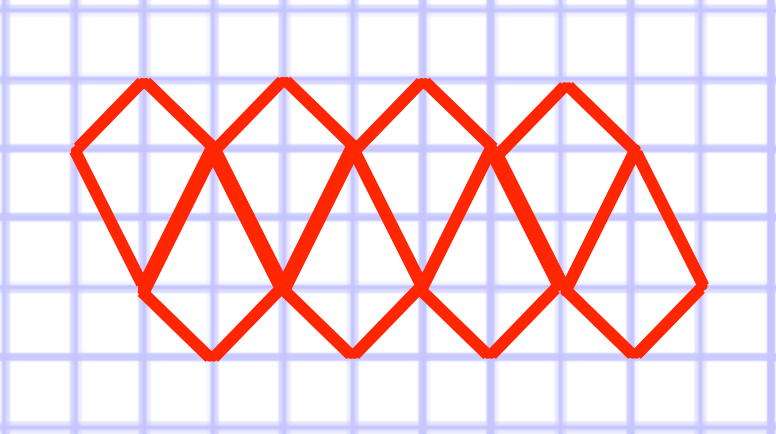Question 1(d)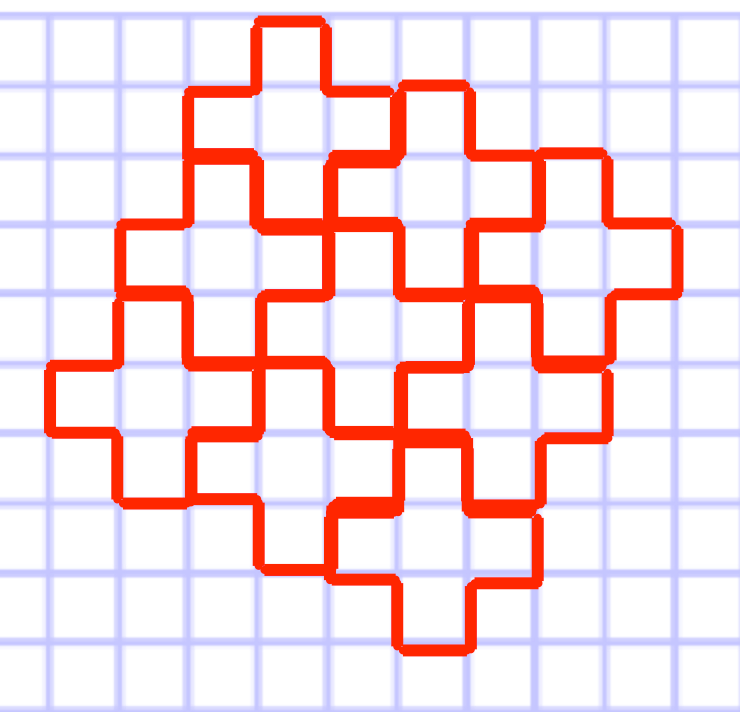Question 1(e)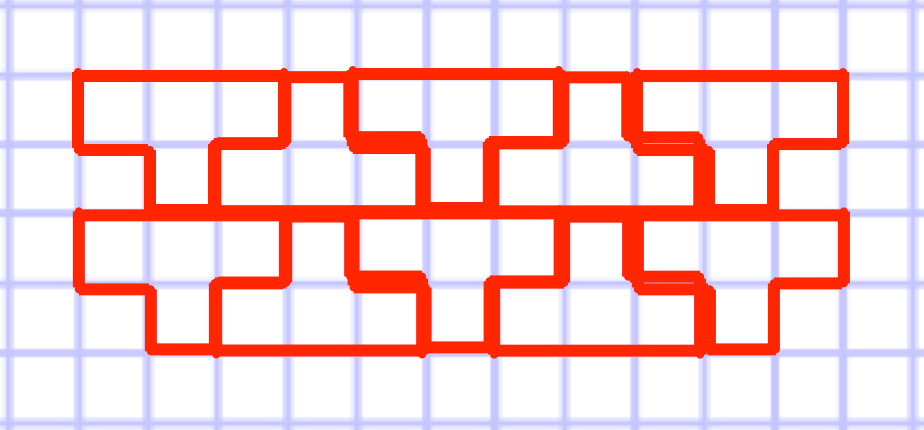Question 1(f)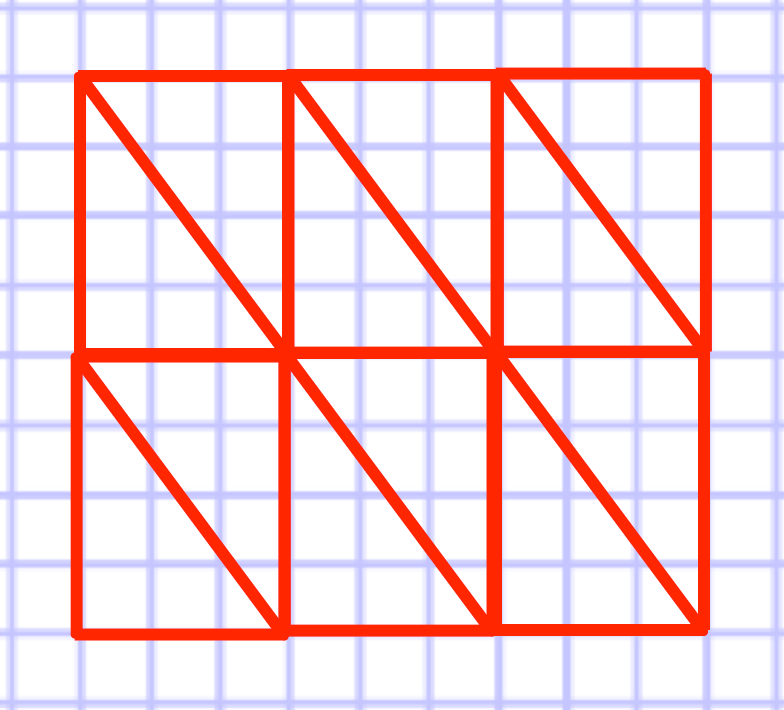Apply

Question 1(a):   60°

Question 1(b):  six identical equilateral triangles will meet at a point and the six 60° angles will make 360°, therefore leaving no gaps.

Question 2(a):  120°

Question 2(b):  three identical regular hexagons will meet at a point and the three 120° angles will make 360°, therefore leaving no gaps.

Question 3(a):  108°

Question 3(b):  135°

Question 3(c):  144°

Question 3(d):  when three identical regular pentagons meet at a point and the three 108° angles will make 324°, therefore leaving a gap. A fourth regular pentagon will not fit in the gap.

Question 3(e): when two identical regular octagons meet at a point and the two 135° angles will make 270°, therefore leaving a gap. A third regular octagon will not fit in the gap.

Question 3(f): when two identical regular decagons meet at a point and the two 144° angles will make 288°, therefore leaving a gap. A third regular decagon will not fit in the gap.

Question 4:

(1)  Dodecagons (2) and Equilateral Triangles

(2)  Dodecagon, Hexagon and Square

(3)  Octagons (2), Square

(4)  Hexagons (2) and Equilateral Triangles (2)

(5)  Hexagon, Squares (2) and Equilateral Triangles (1)

(6)  Hexagon (1) and Equilateral Triangles (4)

(7)  Squares (2) and Equilateral Triangle (3)